Next: Canonical basis Up: Observables and single particle Previous: Observables and single particle

## Hartree-Fock equivalent energies, radii, and nodes of quasiparticle wave functions

For each quasiparticle state, in addition to its quasiparticle energy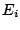given by the HFB equation, one has several other characteristics, which are defined the following way:

• the occupation factor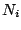which is the norm of the lower component of the HFB quasiparticle wave function;
• the (Hartree-Fock) equivalent energy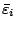which is defined by applying the BCS type formula to the occupation factor, i.e.,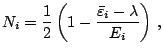(45)

which gives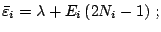(46)

• in the same spirit, the equivalent gap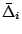is defined by applying the BCS type formula to the HFB quasiparticle energy, i.e.,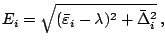(47)

which gives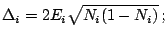(48)

• the rms radius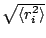is calculated using the lower component of the quasiparticle wave function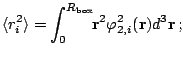(49)

• the number of nodes of the HFB quasiparticle wave function is defined as the number of nodes (including the node at the origin but not the one at infinity) of the component which has the greatest amplitude.
These characteristics of the quasiparticle states can be found in the output file hfb_n_p.spe, see Sec. 7.Next: Canonical basis Up: Observables and single particle Previous: Observables and single particle
Jacek Dobaczewski 2005-01-23